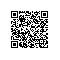# Java中Set集合是如何实现添加元素保证不重复的？

Java中Set集合是如何实现添加元素保证不重复的？

Set集合是一个无序的不可以重复的集合。今天来看一下为什么不可以重复。

Set是一个接口，最常用的实现类就是HashSet，今天我们就拿HashSet为例。

HashSet类实现了Set接口， 其底层其实是包装了一个HashMap去实现的。HashSet采用HashCode算法来存取集合中的元素，因此具有比较好的读取和查找性能。

// 默认构造函数 底层创建一个HashMap
public HashSet() {
// 调用HashMap的默认构造函数，创建map
map = new HashMap<E,Object>();
}

// 带集合的构造函数
public HashSet(Collection<? extends E> c) {
// 创建map。
map = new HashMap<E,Object>(Math.max((int) (c.size()/.75f) + 1, 16));
// 将集合(c)中的全部元素添加到HashSet中
}

// 指定HashSet初始容量和加载因子的构造函数
public HashSet(int initialCapacity, float loadFactor) {
}

// 指定HashSet初始容量的构造函数
public HashSet(int initialCapacity) {
map = new HashMap<E,Object>(initialCapacity);
}

HashSet(int initialCapacity, float loadFactor, boolean dummy) {
}

private transient HashMap<E,Object> map;
// 用来匹配Map中后面的对象的一个虚拟值
private static final Object PRESENT = new Object();

    /**
* 将元素e添加到HashSet中，也就是将元素e作为Key放入HashMap中
*
* @param e 要添加到HashSet中的元素
* @return true 如果不包含该元素
*/
return map.put(e, PRESENT)==null;
}

    public V put(K key, V value) {
// 倒数第二个参数false：表示允许旧值替换
// 最后一个参数true：表示HashMap不处于创建模式
return putVal(hash(key), key, value, false, true);
}

HashMap中的put()方法又调用了putVal()方法来实现功能，再看putVal()的源码。

    final V putVal(int hash, K key, V value, boolean onlyIfAbsent,
boolean evict) {
Node<K, V>[] tab;
Node<K, V> p;
int n, i;
//如果哈希表为空，调用resize()创建一个哈希表，并用变量n记录哈希表长度
if ((tab = table) == null || (n = tab.length) == 0)
n = (tab = resize()).length;
/**
* 如果指定参数hash在表中没有对应的桶，即为没有碰撞
* Hash函数，(n - 1) & hash 计算key将被放置的槽位
* (n - 1) & hash 本质上是hash % n，位运算更快
*/
if ((p = tab[i = (n - 1) & hash]) == null)
//直接将键值对插入到map中即可
tab[i] = newNode(hash, key, value, null);
else {// 桶中已经存在元素
Node<K, V> e;
K k;
// 比较桶中第一个元素(数组中的结点)的hash值相等，key相等
if (p.hash == hash &&
((k = p.key) == key || (key != null && key.equals(k))))
// 将第一个元素赋值给e，用e来记录
e = p;
// 当前桶中无该键值对，且桶是红黑树结构，按照红黑树结构插入
else if (p instanceof TreeNode)
e = ((TreeNode<K, V>) p).putTreeVal(this, tab, hash, key, value);
// 当前桶中无该键值对，且桶是链表结构，按照链表结构插入到尾部
else {
for (int binCount = 0; ; ++binCount) {
// 遍历到链表尾部
if ((e = p.next) == null) {
p.next = newNode(hash, key, value, null);
// 检查链表长度是否达到阈值，达到将该槽位节点组织形式转为红黑树
if (binCount >= TREEIFY_THRESHOLD - 1) // -1 for 1st
treeifyBin(tab, hash);
break;
}
// 链表节点的<key, value>与put操作<key, value>相同时，不做重复操作，跳出循环
if (e.hash == hash &&
((k = e.key) == key || (key != null && key.equals(k))))
break;
p = e;
}
}
// 找到或新建一个key和hashCode与插入元素相等的键值对，进行put操作
if (e != null) { // existing mapping for key
// 记录e的value
V oldValue = e.value;
/**
* onlyIfAbsent为false或旧值为null时，允许替换旧值
* 否则无需替换
*/
if (!onlyIfAbsent || oldValue == null)
e.value = value;
// 访问后回调
afterNodeAccess(e);
// 返回旧值
return oldValue;
}
}
// 更新结构化修改信息
++modCount;
// 键值对数目超过阈值时，进行rehash
if (++size > threshold)
resize();
// 插入后回调
afterNodeInsertion(evict);
return null;
}使用钉钉扫一扫加入圈子
+ 订阅